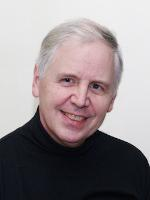# Department of Pure Mathematics and Mathematical StatisticsProfessor of Number Theory and Algebra

Research Interests: Number theory, arithmetic algebraic geometry, modular forms

## Publications

Linear independence in linear systems on elliptic curves
BW Brock, BW Jordan, B Poonen, AJ Scholl, JL Wetherell
– Commentarii Mathematici Helvetici
(2021)
96,
199
Plectic Hodge theory I
J Nekovář, AJ Scholl
(2017)
A Finiteness Theorem for Del Pezzo Surfaces over Algebraic Number Fields
AJ SCHOLL
– Journal of the London Mathematical Society
(2016)
s2-32,
31
On the Hecke algebra of a noncongruence subgroup
AJ Scholl
– Bulletin of the London Mathematical Society
(2016)
29,
395
Introduction to plectic cohomology
J Nekovar, AJ Scholl
– ADVANCES IN THE THEORY OF AUTOMORPHIC FORMS AND THEIR L-FUNCTIONS
(2016)
664,
321
Modular forms, de Rham cohomology and congruences
M Kazalicki, AJ Scholl
(2013)
Hypersurfaces and the Weil Conjectures
AJ Scholl
– International Mathematics Research Notices
(2010)
2011,
1010
On the algebra of modular forms on a congruence subgroup
AJ SCHOLL
– Mathematical Proceedings of the Cambridge Philosophical Society
(2008)
86,
461
Fourier coefficients of Eisenstein series on non-congruence subgroups
AJ SCHOLL
– Mathematical Proceedings of the Cambridge Philosophical Society
(2008)
99,
11
ON SOME $\ell$-ADIC REPRESENTATIONS OF $\mathop{\rm Gal}(\overline{/mathbb{Q}})$ ATTACHED TO NONCONGRUENCE SUBGROUPS
AJ Scholl
– Bulletin of the London Mathematical Society
(2006)
38,
561
• 1 of 4
• >

E1.05

01223 765889